## Basic plotting / visualisation in Python

This is very quick introduction to graphing / visualising in Python done for the Sydney Python course at Arup

Arup Sydney Python Club – Session 8

In :
```from IPython.display import Image
Image(filename='C:/Users/oliver.lock/Desktop/data-visualization-sample.jpg')
```
Out: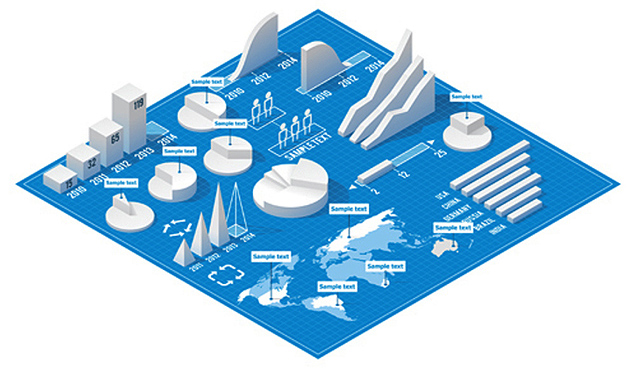Today’s demand for data visualisation tools go beyond the standard charts and graphs used in Excel spreadsheets, displaying data in more sophisticated ways such as infographics, dials and gauges, geographic maps, heat maps, and detailed bar, pie and rose charts. This demand extends to visualisations with interactive capabilities – there is an expectation that we are able to create and can allow manipulation/drilling into the data for querying and analysis.

Using scripting / programmatic techniques for our data analysis and visualisation means we are able to surpass a number of barriers formerly in software packages (again, Excel), especially when faced with issues to do with speed, display, customisation and data size. Python has several options for creating both static and interactive data analysis/visualisation environments.

The Python scientific stack (http://scikit-learn.org/stable/) is fairly mature, and there are libraries for a variety of use cases, including machine learning http://scikit-learn.org/stable/), network analysis (https://networkx.github.io/) and data analysis (http://pandas.pydata.org/).

Many new Python data visualization libraries have been created in the past few years to close the gap. This class will focus on using ‘matplotlib’, one of the many libraries available. Some alternate libraries will be explained below.

# What is Matplotlib?¶

Simply put, matplotlib is a graphing library for Python. It has an array of tools that you can use to create anything from simple scatter plots, to sin curves, to 3D graphs. It is used heavily in the scientific Python community for data visualisation. It is designed to closely resemble MATLAB (which can be found in many engineering courses).

You can read more about the ideas behind matplotlib on their website, but I especially recommend taking a look at their gallery to see the amazing things you can pull off with this library.

http://matplotlib.org/gallery.html

# What else is out there?¶

Some of the many alternatives to matplotlib include seaborn, ggplot and plotly.

Seaborn; The main difference between matplotlib and seaborn is its default styles and palettes, which are more aesthetically pleasing and modern. Seaborn is built on top of matplotlib, so it doesn’t hurt to learn this first.

ggplot; ggplot is based on ggplot2 in stats package ‘R’ and concepts from the ‘Grammar of Graphics’. The outputs are much easier to create and much better looking, but it isn’t designed for high levels of customisation. It sacrifices complexity for a simple method of plotting (kind of like iOS vs Android). One of the advantages is its close integration with ‘pandas’ – a popular data analysis library. If you are familiar with R or SQL it might be useful to look at this.

Plotly; Plotly is an online platform for data visualisation, and within Python one can create interactive graphs and charts. Plotly’s forte is making interactive plots and offers some charts you won’t find in most libraries, like contour plots, dendograms, and 3D charts. If you are thinking of making something interactive or for the web with your Python code, this would be worth investigating.

# Some examples¶

In :
```# Bus network analysis with San Francisco 7 terabyte data set spanning ~2008 - 2014.
# Below extracts are from a tool that allows quick graphing and mapping
# / visualisations of any time span, at stop,route and network level for the very large dataset generated from the
# bus positioning and passenger countring system.
# See: http://pandas.pydata.org/pandas-docs/stable/visualization.html

from IPython.display import Image
```
Out: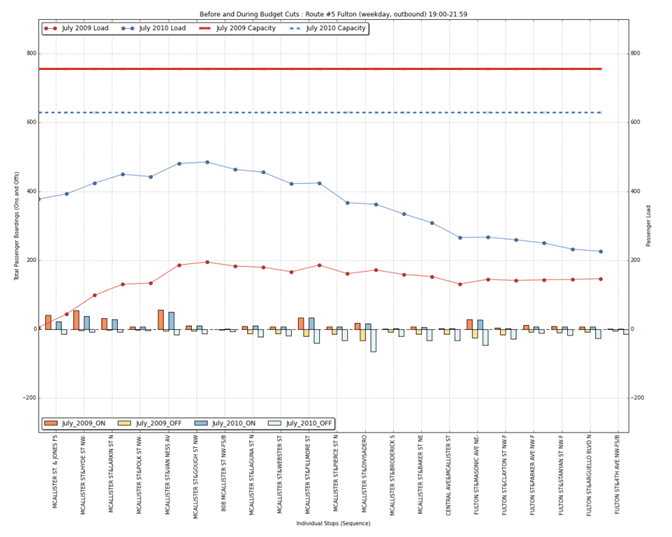In :
```#This combines the bus data with analystical package 'networkx' to study the network according to graph theory
# Generates visualisation of specified time period and how network stops perform
#See: https://networkx.github.io/

from IPython.display import Image
Image(filename='C:/Users/oliver.lock/Desktop/bus_graph_theory_degree.png')
```
Out: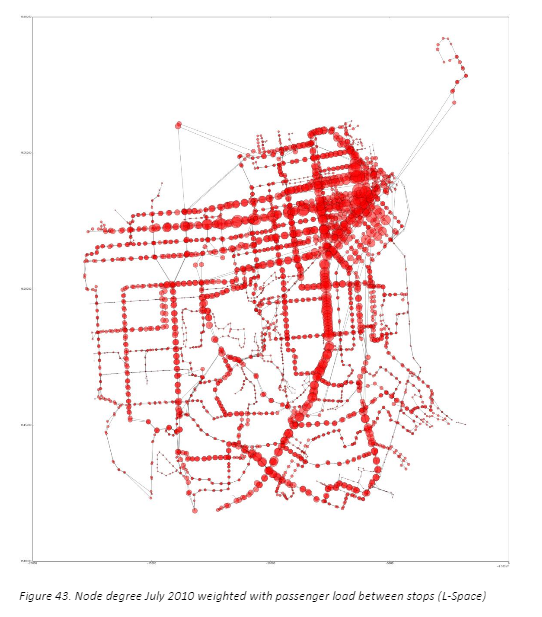# #plotly example¶

https://plot.ly/python/bubble-charts/

# What makes a good data visualisation?¶

In his 1983 book The Visual Display of Quantitative Information, Edward Tufte defines ‘graphical displays’ and principles for effective graphical display. Many of these ideas have stuck in the industry to date and the below principles are a staple 101 to data visualisation.

A good data visualisation should:

1. Show the data
2. Provoke thought about the subject at hand
3. Avoid distorting the data
4. Present many numbers in a small space
5. Make large datasets coherent
6. Encourage eyes to compare data (Interactivity)
7. Reveal data at several levels of detail
8. Serve a reasonably clear purpose
9. Be closely integrated with statistical and verbal descriptions of the dataset

# Section 1 – Line Graphs¶

## 1A ) Nailing the basic line graph¶

### Start by importing these Python modules¶

In :
```import matplotlib.pyplot as plt
import numpy as np
%matplotlib inline
```

### Generate some data¶

##### For example here we have given some values to the variable X, in a list and values to the variable Y. The order is important here!¶
In :
```x = [1,2,3,4,5,6,7,8,9,10]
y = [1,2,4,8,16,32,64,128,256,512]
```

### Plot a graph¶

In :
```#plot with all default settings
plt.plot(x,y)
#! plt.show()
```
Out:
`[<matplotlib.lines.Line2D at 0xe88bdd8>]`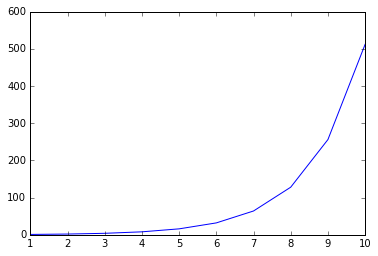In :
```#flip x and y axis
plt.plot(y,x)
#! plt.show()
```
Out:
`[<matplotlib.lines.Line2D at 0xe689588>]`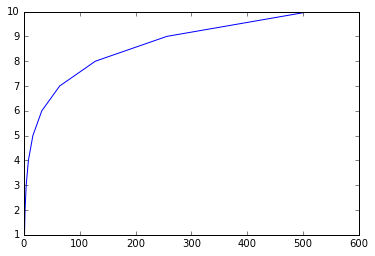In :
```# red dashed line
plt.plot(x,y,'r--')
#!plt.show()
```
Out:
`[<matplotlib.lines.Line2D at 0xeb59c18>]`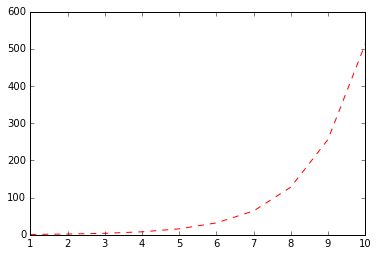## Scatter plot¶

In :
```#a line plot can easily become a scatter plot by changing markers
#plot with blue markers
plt.plot(x,y,'bo')
#!plt.show()
```
Out:
`[<matplotlib.lines.Line2D at 0xed74320>]`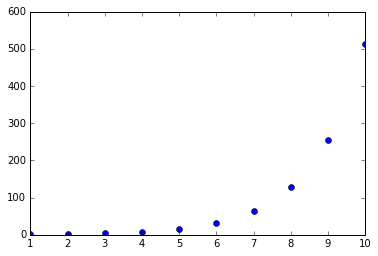In :
```#Two datasets, same plot
x1 = [1,2,3,4,5,6,7,8,9,10]
y1 = [1,2,4,8,16,32,64,128,256,512]
x2 = [1,2,3,4,5,6,7,8,9,10,11]
y2 = [10,20,40,89,160,300,640,450,500,510,700]
plt.plot(x1,y1,x2,y2,marker='o',linestyle='None')
#!plt.show()
```
Out:
```[<matplotlib.lines.Line2D at 0xefc9be0>,
<matplotlib.lines.Line2D at 0xefc9d68>]```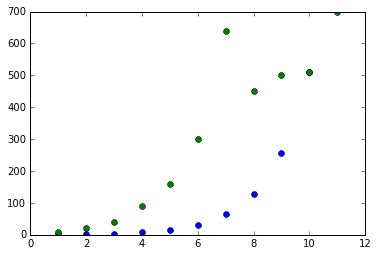In :
```#More Control, plotting more than one dataset with alternate styling
x1 = [1,2,3,4,5,6,7,8,9,10,11]
y1 = [1,2,4,8,16,32,64,128,256,512,1024]
x2 = [1,2,3,4,5,6,7,8,9,10,11]
y2 = [10,20,40,89,160,300,640,450,500,510,700]
plt.plot(x1,y1, 'go-', label='Expected', linewidth=2)
plt.plot(x2,y2, 'rs',  label='Sampled')
plt.axis([0, 12, 0, 800])
plt.legend(loc='best')
#!plt.show()
```
Out:
`<matplotlib.legend.Legend at 0xf1a9748>`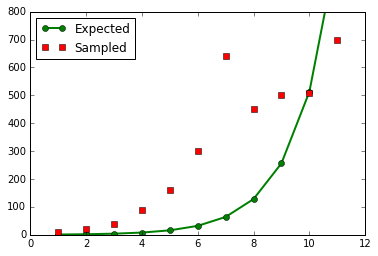In :
```#if you run this command if will give you documentation on styles etc
#!help(plt)
```

## Bar chart¶

In :
```#example bar chart with positive and negative values

import numpy as np
import matplotlib.pyplot as plt

n = 12

X = np.arange(n)

Y1 = (1-X/float(n)) * np.random.uniform(0.5,1.0,n)
Y2 = (1-X/float(n)) * np.random.uniform(0.5,1.0,n)

plt.bar(X, +Y1, facecolor='#9999ff', edgecolor='white')
plt.bar(X, -Y2, facecolor='#ff9999', edgecolor='white')

#!plt.show()
```
Out:
`<Container object of 12 artists>`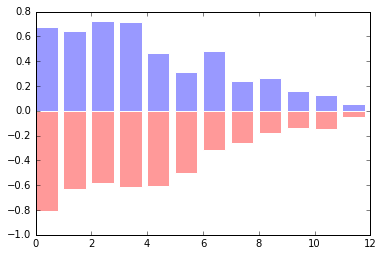## 1B ) Tidying / extending our graphs¶

##### Now we can extend this further by looking at some other aspects of a chart, with more sophisticated data¶

In :
```#Create a range of dates
x = range(1940,2016)
#Create a list of a fluctuating random value the same length of that date range
y = np.random.randn(len(x)).cumsum()
```

### Plot the data¶

In :
```plt.plot(x, y)
#!plt.show()
```
Out:
`[<matplotlib.lines.Line2D at 0xf595588>]`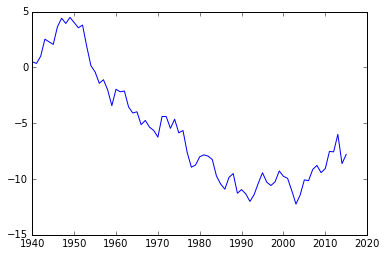### Add titles / legends / symbology¶

In :
```plt.title('Fake Global Sea Temperature over Time')

plt.xlabel('Date')

plt.ylabel('Sea Temperature')

plt.grid(True)

plt.figtext(0.995, 0.01, 'Data Source: Bureau of Astrology, 2016', ha='right', va='bottom')

plt.legend(loc='best', framealpha=0.5,  prop={'size':'small'})

plt.gcf().set_size_inches(8, 4)

plt.plot(x, y)

#!plt.show()
```
Out:
`[<matplotlib.lines.Line2D at 0xeee50f0>]`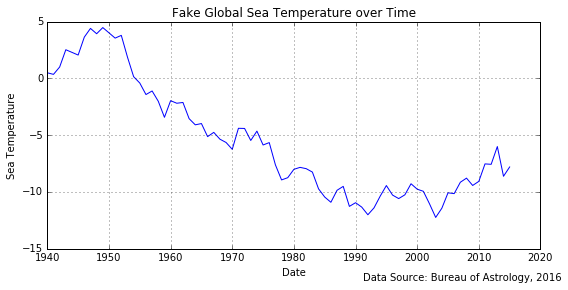# Exercises¶

After your previous exercises with similar weather station data, you have become a well-known international expert.

You have been requested to produce the following visualisations.

The data can be found here:

https://github.com/oliverclock/pythonviz/blob/master/weather_places.csv

1) Produce an image of all of the temperature or rainfall values (regardless of geographic data)

2) Produce an image that explains the potential relationship between latitude and rainfall

3) Produce an image which shows the countries in their geographic location (using supplied latitude/longitude field)

4) Produce the previous image this time with the plot points coloured or scaled by the temperature attribute

5) Extension – Visualise these values on a world map basemap

6) Extension – Visualise these values as a choropleth map (i.e. a map that shows country shapes rather than just points)

Hints: